Wang, W., Sun, L., Wu, M., Li, X., and Song, W. (2020). "Molecular dynamics simulation of bamboo heat treatment with cellulose based on molecular different weight fractions of water," BioRes. 15(3), 6766-6780.

#### Abstract

Eight groups of cellulose amorphous region models in which the mass fraction of water was separately 0%, 1%, 2%, 3%, 4%, 5%, 6%, and 7% were established using a molecular dynamics software material studio. The PCFF force field was selected to simulate the molecular dynamics of the model under the constant-pressure and constant-temperature (NPT) ensemble. The simulated temperature was set to 433.15 K. The experiment showed that the hydrogen bonds between cellulose chains affected the structure of cellulose, which led to the change of the end-to-end distance of the cellulose chain and the overall size of a cell. The diffusion degree of water molecules was closely related to the number of hydrogen bonds between cellulose and water. In the process of heat treatment of bamboo, the present simulation results suggest that the structure of bamboo may be damaged when the mass fraction of water vapor reaches or exceeds 7%.

Molecular Dynamics Simulation of Bamboo Heat Treatment with Cellulose Based on Molecular Different Weight Fractions of Water

Wei Wang,a,* Liyue Sun,a Mingshuai Wu,a Xing Li,a and Wenlong Song b

Eight groups of cellulose amorphous region models in which the mass fraction of water was separately 0%, 1%, 2%, 3%, 4%, 5%, 6%, and 7% were established using a molecular dynamics software material studio. The PCFF force field was selected to simulate the molecular dynamics of the model under the constant-pressure and constant-temperature (NPT) ensemble. The simulated temperature was set to 433.15 K. The experiment showed that the hydrogen bonds between cellulose chains affected the structure of cellulose, which led to the change of the end-to-end distance of the cellulose chain and the overall size of a cell. The diffusion degree of water molecules was closely related to the number of hydrogen bonds between cellulose and water. In the process of heat treatment of bamboo, the present simulation results suggest that the structure of bamboo may be damaged when the mass fraction of water vapor reaches or exceeds 7%.

Keywords: Molecular dynamics; Mechanical properties; Heat treatment; Mass fraction of water

Contact information: a: College of Engineering and Technology, Northeast Forestry University, Harbin, 150040, China; b: College of Mechanical and Electrical Engineering, Northeast Forestry University, Harbin, 150040, China; *Corresponding author: cedar3@163.com

INTRODUCTION

Bamboo is an important renewable resource. Compared with wood, it has the characteristics of high strength, toughness, rigidity, and easy longitudinal division (Li et al. 2015). Bamboo heat treatment is a technique in which bamboo is placed in a heat transfer medium, such as air, inert gas, or water vapor, and subjected to short-term pyrolysis treatment at a high temperature of 160 °C to 250 °C. The heat treatment of bamboo in water vapor plays an important role in improving the utilization and quality of bamboo because of the change of the internal structure of the bamboo material. The water absorption following treatment is decreased, and the dimensional stability increased, which can help to improve the stability of bamboo in a humid environment (Cao et al. 2012; Wang et al. 2018). The study found that the mass fraction of water vapor during heat treatment has a significant impact on the properties of bamboo after heat treatment.

Cellulose is the main component of bamboo that determines its structure and properties. Cellulose-based materials are also among the most important raw materials in industry. Researches have noted that every 1% increase in moisture content will double the rate of cellulose hydrolysis and aging in the operating environment of transformers (Lundgaard et al. 2004). Since the hydrolytic aging of cellulosic material is closely related to the moisture content and distribution, we consider that during the heat treatment of bamboo, the mass fraction of water vapor should not be too high, as that might be expected to reduce the mechanical properties of bamboo.

Cellulose is a polymeric compound formed by polymerization of D-glucopyranose. Natural cellulose consists of crystalline and amorphous regions. The cellulose molecules in the crystalline zones are arranged in a compact and orderly manner. In the process of heat treatment with steam, the action of water molecules is limited to the surface of the crystallized region, and it cannot interact well with the crystalline zone (LeNeveu et al. 1976; Lee and Rossky 1994; Heiner et al. 1998). However, cellulose molecules in the amorphous region are irregularly distributed, and thus the adsorption of water molecules is strong. During heat treatment of cellulose in the presence of water, water molecules enter the cellulose amorphous region. Structural changes of cellulose occur, further affecting the mechanical properties of cellulose. Therefore, this paper selects the amorphous region as the object to study the variation of bamboo in the heat treatment process.

The repeating unit of natural cellulose is 𝛽-D-glucose. A cellulose chain passes through several crystal regions and amorphous regions. The length of the amorphous region in the cellulose chain is really small. When studying the properties of cellulose amorphous regions, Mazwau and Heux (2003) used cellulose chains with different degrees of polymerization (DP) to build an amorphous cellulose model. After a simulation experiment, it was found that there is no obvious difference in molecular conception or physical and chemical properties. Considering DP=40 cellulose looks closer to nature, they chose DP=40 cellulose chain to build an amorphous cellulose model. When studying the mechanical properties of amorphous cellulose, Chen et al. (2004) used a DP=20 cellulose chain to construct the amorphous region, and the obtained mechanical properties were basically consistent with the experimental values. Since the object of the present work is to study the effect of different moisture content on the mechanical properties of cellulose during the heat treatment of bamboo, DP=20 was used as the length of the cellulose chain to find the suitable range of water vapor mass fraction during heat treatment of bamboo.

EXPERIMENTAL

Methods

Computational details

Molecular dynamics simulations were performed using Materials Studio software (Materials Studio, BIOVIA, v.8.0, San Diego, CA, USA). The amorphous region of cellulose was established using an amorphous polymer construction method (Theodorou and Suter 1985). Because the degree of polymerization has no remarkable effect on the physicochemical properties of the amorphous region of cellulose, a cellulose chain with a degree of polymerization of 20 was selected (Mazeau and Heux 2003). The change in cellulose in different mass fractions of water vapor was modeled using the Amorphous Cell (AC) Tools module. The PCFF force field was selected because it is suitable for the calculation of organic matter, like the cellulose chain and water vapor mixing system (Sun et al. 2018), the charge addition method uses the Ewald method; the Van der Waals addition method is an atom-based method. The target density was set to 1.5 g/cm3 (Onyon 1972) to establish a water vapor-cellulose model.

The mass fraction of water vapor is 0%, 1%, 2%, 3%, 4%, 5%, 6%, and 7%, respectively. There was a cellulose molecular chain with a degree of polymerization of 20 in the model without water; 4 water vapor molecules were added and a cellulose molecular chain (DP = 20) in the model with 1% water; 8 water vapor molecules were added and a cellulose molecular chain (DP = 20) in the model with 2% water; 12 water vapor molecules were added and a cellulose molecular chain (DP = 20) in the model with 3% water; 16 water vapor molecules were added and a cellulose molecular chain (DP = 20) in the model with 4% water; 20 water vapor molecules were added and a cellulose molecular chain (DP = 20) in the model with 5% water; 24 water vapor molecules were added and a cellulose molecular chain (DP = 20) in the model with 6% water; 29 water vapor molecules and a cellulose molecular chain (DP = 20) were added to the model with 7% water. The simulated unit cell size was 19.6 × 19.6 × 19.6 (A3).

In Fig. 1, pink shows a cellulose molecular chain, and blue shows water molecules.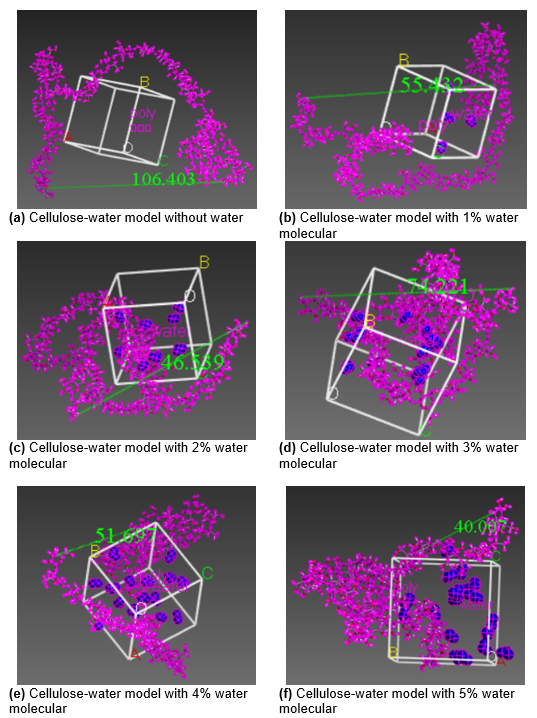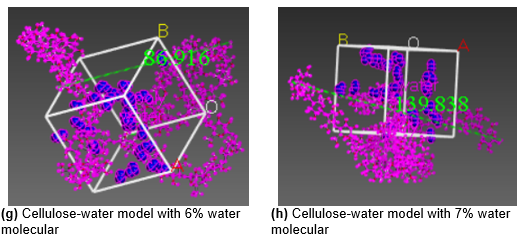Fig. 1. Modeled microstructures

Dynamic simulation

After the cellulose-water system model was obtained (shown in Fig. 1), the preliminary geometry optimization was performed using the calculation in the Forcite module, selecting the PCFF force field, and using the smart algorithm to calculate 1000000 steps, so the free motion of the mixed system molecules tend to balance and achieve energy minimization. Then, the model was simulated in 433.15 K in an isothermal-isobaric pressure ensemble (NPT). The initial velocity was set to random; the time step was 1 fs; the total length was 1000 ps, and the temperature control method was selected by Andersen (Zhang et al. 2013).

The Berendsen method (Iwona and Lea 2013) was used to control pressure; the electronic contribution was computed using the Ewald method (Ewald 1921), and the Van der Waals force was computed by atom-based calculations (Anderson 1980).

RESULTS AND DISCUSSION

The balance of the system

In the molecular dynamics (MD) simulation, temperature and energy were used as the basis of system equilibrium, as shown in Figs. 2 and 3 (the cellulose-water system model with 1% water as an example).

When the fluctuation range of the value was between 5% and 10%, the system equilibrium was determined. The simulation accuracy can also be described by energy convergence parameters, which are defined as follows (Eq. 1),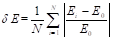(1)

where the parameter N refers to the total number of steps simulated. The parameter E0 refers to the initial energy of the simulation system; the parameter Ei refers to the energy of the system after i steps. When δE ≤ 0.001 ~ 0.003, the simulation results are reliable.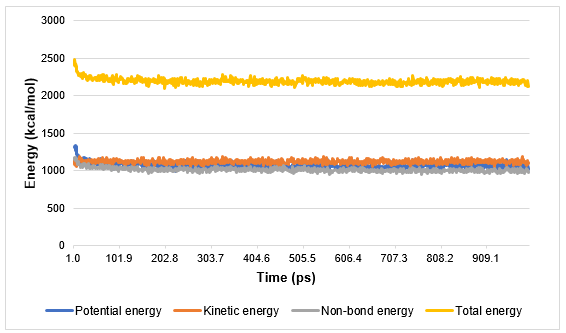Fig. 2. Energy-time change graph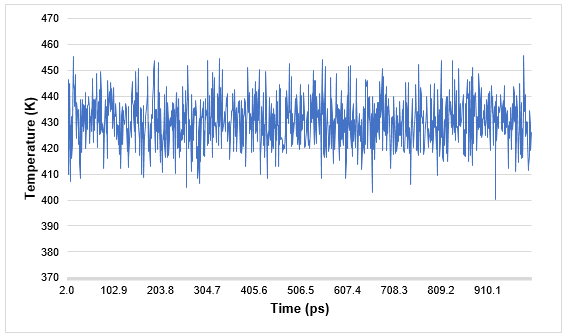Fig. 3. Temperature-time change diagram

The MD simulation of the water molecule-cellulose mixed system with each mass fraction shows that the energy fluctuation of 100 ps was stable, and the fluctuating value and energy convergence parameters of the mixed system with each mass fraction of water molecule were as shown in Table 1. The energy fluctuation values of the last 100 ps were between 0.6% to 4.2%, the energy convergence parameters were between 0.0013 and 0.0020. Therefore, after 1 ns molecular dynamics simulation, it can be considered that the system tends to be balanced and the simulation results are reliable.

Table 1. Equilibrium Parameter Values of Cellulose-water System Under Different Mass Fractions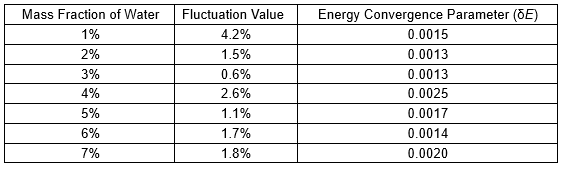Lattice parameters

The cell formed by the cellulose-water system is a cube, and the cell parameters represent the length of the cell cube to describe the cell size. In this paper, the cellulose-water models all contained a cellulose chain (DP = 20) and water molecules with mass fractions of 0% ,1 %, 2%, 3%, 4%, 5%, 6%, and 7%.

After the molecular dynamic simulation, the cell parameters of cellulose-water, which are the length, width and height of the cell cube of cellulose-water molecules, are shown in the Table 2.

Table 2. Cell Parameters of Cellulose-water Model Under Different Mass Fraction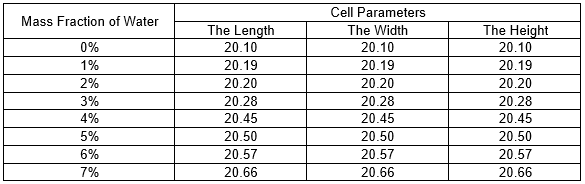From the data in the Table 2, the length of the lattice increased from 20.10 to 20.66 with the water molecules added. From Fig. 4, it can be clearly seen that with the increase of the mass fraction of water, the overall cell size of the cellulose-water model showed an upward trend (dotted line is the trendline), which proved that the water absorption of the cellulose chain was enhanced.

The number of hydrogen bonds

The properties of cellulose are mainly due to the high degree of polymerization of cellulose and the numerous hydrogen bonds among cellulose molecules (Hinterstoisser et al. 2003). The heat treatment process of the cellulose-water system can be analyzed by the Chain Flexibility theory (mainly hydrogen bond action). Adding water molecules to the cellulose chain structure will destroy the hydrogen bond between the original chains of cellulose and form the hydrogen bond between the cellulose chain and water.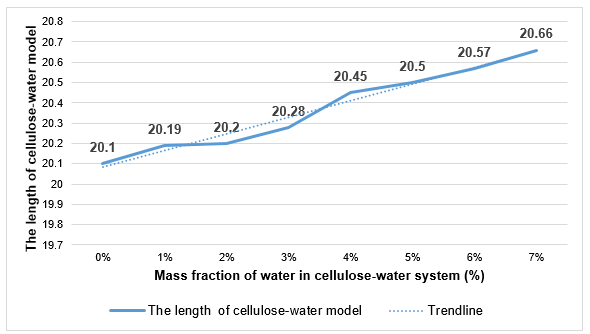Fig. 4. Variation of cell size with the mass fraction of water in cellulose-water model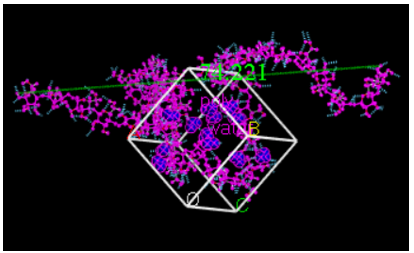Fig. 5. The hydrogen bonds in the model

The hydrogen bonds in the cellulose-water system model were divided into intermolecular hydrogen bonds and intramolecular hydrogen bonds (shown as Fig. 5). Intramolecular hydrogen bonds refer to the hydrogen bonds formed in the cellulose chain, and the intermolecular hydrogen bonds refer to the hydrogen bonds formed between the cellulose chain and the water molecule. Intramolecular hydrogen bonds play an important role in the thermal stability and mechanical properties of cellulose molecules. Table 3 shows the number of hydrogen bonds in the cellulose-water system. It can be seen from Table 3 that the number of hydrogen bonds between cellulose molecules was between 100 and 142, and the number of hydrogen bonds between cellulose and water molecules did not exceed 12. Thus, there were more hydrogen bonds between cellulose molecules than intramolecular hydrogen bonds between the cellulose chain and water molecules in each cellulose-water system model with different water content, which fully indicated that the hydrogen bonds between cellulose chains played an important role in maintaining the stability of the cellulose structure and mechanical properties.

Figure 6 shows the trend diagram of all kinds of hydrogen bonds. The good performance of cellulose is mainly due to the high degree of polymerization of cellulose and the numerous hydrogen bonds in cellulose. The number of hydrogen bonds between cellulose chains increased at first from 130 to 142, showing that a small number of water molecules made more hydrogen bonds inside the cellulose molecules, causing the cellulose molecules to gain more excellent mechanical properties. And then the number of hydrogen bonds decreased to 120, indicating that while too much water added they would destroy the structure of cellulose chains and caused aging degradation of bamboo. This also confirmed the change of the trend of the end-to-end distance of cellulose shortening and then increasing.

Table 3. Number of Hydrogen Bonds in the Cellulose-water System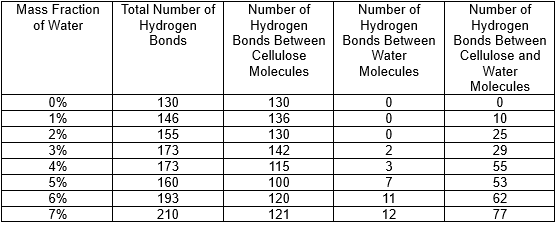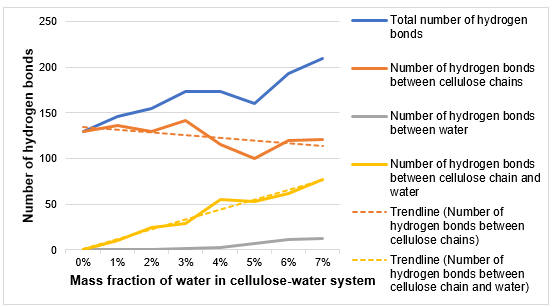Fig. 6. The number of hydrogen bonds in cellulose-water system

The mean square displacement and the molecular diffusion coefficient

The mean square displacement (MSD) is the mean squared sum of the position vector increments of a gas molecule after a simulation time in the system. The MSD can effectively characterize the migration path and behavior of molecules and plays a role in understanding the diffusion changes of gas molecules. It is represented by Eq. 2,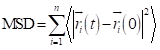(2)

where < > indicates the average value, which indicates the centroid displacement of the i-th particle after a certain simulation time and the particle at the initial time, and n is the number of diffusion particles. The molecular diffusion coefficient D is often used to describe the diffusion behavior of small gas molecules. The expression is: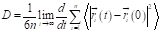(3)

It can be further simplified as Eq. 4,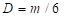(4)

where m is the slope obtained by fitting the MSD curve using the least squares method.

Figure 7 shows the MSD of water vapor molecules in a model with different mass fractions of water molecules. The MSD curve of water vapor molecules in different models in Fig. 6 was fitted to obtain the slope m and the goodness of fit, and the gas small molecule diffusion coefficient D of each model was calculated according to Eq. 4 as shown in Table 4.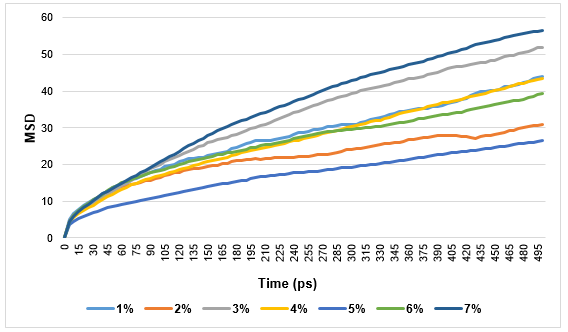Fig. 7. Mean square displacement of water molecules

Table 4. Diffusion Coefficient of Water Molecules in Cellulose-water System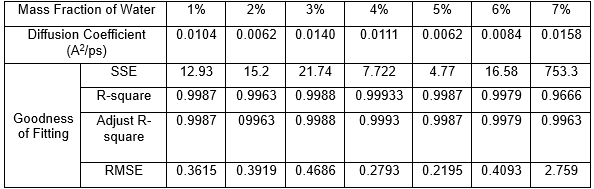Where SSE is sum of squares due to error, and RMSE is root mean square error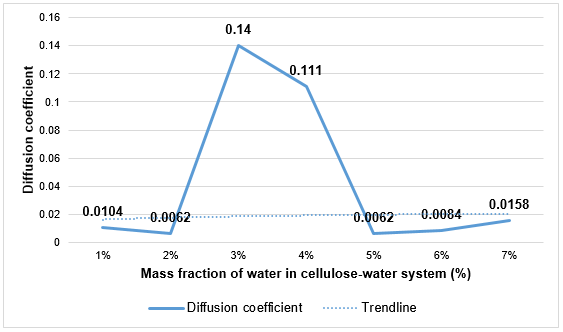Fig. 8. Diffusion coefficient of water molecules in cellulose-water system

It can be seen from Fig. 8 and Table 4 that the overall trend of the diffusion coefficient of water molecules increased from 0.0104 to 0.0158, but there was an abnormal value when the mass fraction of water was 3% and 4%. The reason for this could have been that when the mass fraction of the water molecule was only 1% or 2%, the Van der Waals force between the cellulose and water molecules could adsorb water molecules. However, when the mass fraction of the water molecules reached 3%, the Van der Waals force between the molecules was not sufficient to attract the water molecules due to the increase of the water molecule content, and the number of hydrogen bonds was not noticeable, so the diffusion of water molecules was obviously enhanced. When the mass fraction of water molecules was as large as 5%, the number of hydrogen bonds increased so that the hydrogen bonds played a role in attracting the water molecules again, so that the diffusion coefficient was again reduced to 0.0062.

End-to-end distance

The more flexible sugar linkages in the cellulose molecule determine the flexibility of the cellulosic material. The length between the chain end and the end of the cellulose molecule will vary from time-to-time due to the thermal motion of the molecule. The end-to-end distance, which is the distance between the leading end and the end of the chain, describes the degree of aggregation and contraction of the chain. The end-to-end distance is an important parameter to measure the thermal motion of cellulose molecules. Table 5 shows the end-to-end distance range of cellulose molecules under different water molecular mass fractions. If the end-to-end distance of the cellulose molecular chain changes greatly, it means that the molecular structure inside the cellulose material changes seriously. Figure 9 shows the change trend of the end distance of the cellulose chain, which decreased at first from 109.4 to 48.7 and then increased from 47 to 144.2, which was consistent with the change trend of the hydrogen bond number between cellulose chains. The abnormal value appeared when the mass fraction of water was 3%. It is speculated that it may have been caused by the force between cellulose molecules and water molecules just like the change of the diffusion coefficient of water molecules.

Table 5. The End-to-end Distance in the Cellulose-water System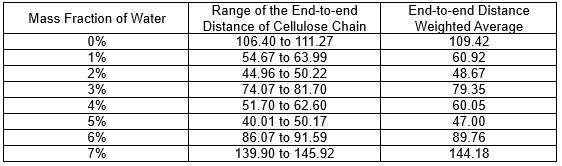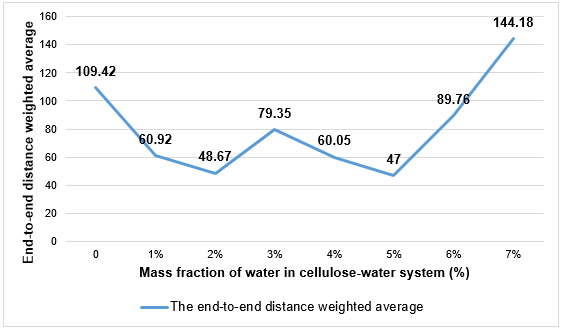Fig. 9. The end-to-end distance of the cellulose chain

Mechanical property

The kinetic simulation results at 160 °C were calculated and the mechanical parameters obtained were able to characterize the mechanical properties of the bamboo. Because the amorphous region of cellulose can be considered isotropic, its mechanical properties can be calculated by C11 and C12, so that Eq. 5,

λ = C12 (5)

and Eq. 6,

μ = (C11 – C12) / 2 (6)

where λ and μ are called Lame constants. The value of Cij is given by Eq. 7: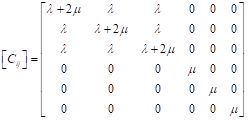(7)

The Lame constant is used to calculate mechanical properties such as Young’s modulus E, shear modulus G, bulk modulus K, and Poisson’s ratio γ. The formula is as follows (Eq. 8):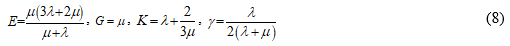The mechanical properties of the cellulose-water system model under different mass fractions of water were obtained by MD calculation, as shown in Table 6. Young’s modulus is the ratio of the longitudinal stress to strain of the material, that is, the longitudinal elastic modulus, which measures the rigidity of the material, and its performance is proportional to the numerical value. The shear modulus is the ratio of shear stress to strain; and the bulk modulus is a measure of the incompressibility of a solid material. Poisson’s ratio is the ratio of transverse stress to strain, that is, the transverse elastic modulus, characterizing the plasticity of the material.

Table 6. Mechanical Properties of Cellulose-water System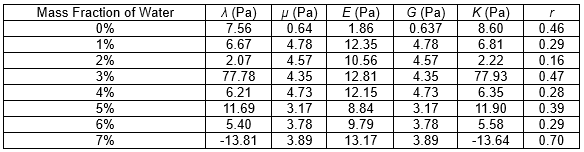It can be seen from Table 6 and Fig. 10 that with the increase of the mass fraction of water, the Young modulus (E), shear modulus (G), and Poisson’s ratio (r) of a cellulose chain did not change obviously, but the volume modulus (K) had a remarkable change. When the mass fraction of water was 3%, it increased greatly at 77.78, which led to the increase of the volume modulus due to the large number of hydrogen bonds between cellulose chains and the compact structure. When the mass fraction of water reached 7%, the volume modulus became -13.81 (to be negative), which is impossible in practical applications. The structure of bamboo was seriously destroyed when the mass fraction of water reached 7%. This conclusion was in good agreement with the end-distance of the cellulose chain and the diffusion coefficient of water molecules. It is also consistent with the conclusion that water will age and hydrolyze cellulose, which Lungfaard et al. (2004) studied earlier.